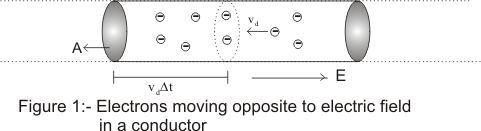Drift velocity and relation between drift velocity and electric current

(3) Drift Velocity

• Metallic conductors have large numbers of elctrons free to move about.These elctrons which are free to move are called conduction electrons
• Thus valence electrons of atom becomes the conduction electrons of the metals
• At room temperature,these conduction electrons moves randomly inside the conductor more or less like a gas molecule
• During motion,these conduction electrons collide with ions(remaining positive charged atom after the valence electrons move away) again and again and there direction of motion changes after each and every collision.
• As a results of these collisions atoms moves in a zig-zag path
• Since in a conductor there are large number of elctrons moving randomly inside the conductor.Hence they have not net motion in any particular direction.Since the number of electrons crossing an imaginary area ΔA from left to right inside the conductor very nearly equals the number of electron crossing the same area element from right to left in a given interval of time leaving flow of electric current through that area nearly equals to zero
• Now when we applied some P.D using a battery across the two ends of the conductor,then an electric field sets up inside the conductor
• As a result of this electric field setup inside the conductor,conduction electron expeirence a force in direction opposite to electric field and this force accelerates the motions of the electrons
• As a result of this accelerated motion electrons drifts slowly along the length of the conductor towards the end at higher potential
• Due to this acceleration velocity of electron's increases only for short interval of time as each acclerated electrons suffers frequent collision with positive ions and looses their Kinectic energy
• After each collision electrons starts fresh in random direction ,again get accelerated and loose their gained Kinetic energy in another collision
• This extra velocity gained by the electrons is lost in subsequent collison and the processes continued till the electron reach positive end of the conductor
• Under the effect of electric field inside the conductor ,free electrons have random thermal velocities due to the room tmeperature and small velocities with which they drift towards the positive end of the conductor.
• if τ is the average time between two successive collisions and E is the strength of applied electric field then force on electron due to applied electric field is
F=eE
Where e is the amount of charge on electron
• if m is the mass of electron ,then acceleration produced is given by
a=eE/m
• Since electron is acclerated for an average time interval τ,additional velocity acquired by the electron is
vd=aτ
or vd=(eE/m)τ                    (3)
This small velocity imposed on the random motion of electrons in a conductor on the application of electric field is known as drift velocity
• This drift velocity is defined as the velocity with which free electrons gets drifted towards the positive end of the conductor under the influence of externally applied electric field

(4) Relation between drift velocity and electric current

• Consider a conducting wire of lenghth L and having uniform cross-section area A in which electric field is present• Consider in the wire that there are n free electrons per unit volume moving with the drift velocity vd
• In the time interval Δt each electron advances by a distance vdΔt and volume of this portion is AvdΔt and no of free electron in this portion is nAvdΔt and all these electrons crosses the area A in time Δt
• Hence charge crossing the area in time Δt is
ΔQ=neAvdΔt
or
I=ΔQ/Δt =neAvd                    (4)
This is the relation between the electric current and drift velocity
• If the moving charge carriers are positive rather than negative then electric field force on charge carriers would be in a direction of electric fields direction and drift velocity would be in left to right direction opposite to what shown in fig-1
• In terms of drift velocity current density is given as
j=I/A=nevd                       (5)